﻿ 变分数阶扩散方程微分阶数的数值反演 Numerical Inversion for the Fractional Order in the Variable-Order Time-Fractional Diffusion Equation

Advances in Applied Mathematics
Vol.04 No.04(2015), Article ID:16356,10 pages
10.12677/AAM.2015.44041

Numerical Inversion for the Fractional Order in the Variable-Order Time-Fractional Diffusion Equation

Di Liu, Chunlong Sun, Gongsheng Li*, Xianzheng Jia

*通讯作者。

School of Sciences, Shandong University of Technology, Zibo Shandong

Received: Oct. 22nd, 2015; accepted: Nov. 7th, 2015; published: Nov. 12th, 2015

Copyright © 2015 by authors and Hans Publishers Inc.

This work is licensed under the Creative Commons Attribution International License (CC BY).

http://creativecommons.org/licenses/by/4.0/ABSTRACT

An implicit finite difference scheme is introduced to solve the variable-order time-fractional diffusion equation, and an inverse problem of determining the variable fractional order is set forth using the additional measurements at one interior point. The homotopy regularization algorithm is applied to solve the inverse problem, and numerical examples are presented. The computational and inversion results demonstrate that the variable order has important influence on the problem, and that the computations become effective when the variable order goes to 1.

Keywords:Variable-Order Fractional Diffusion Equation, Inverse Problem, Homotopy Regularization Algorithm, Numerical Inversion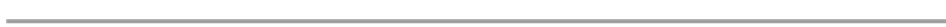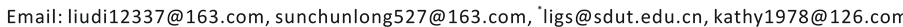1. 引言

2. 正问题及其数值求解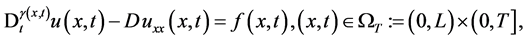(1)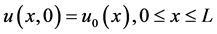， (2)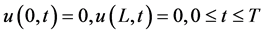， (3)， (4)

2.1. 差分格式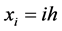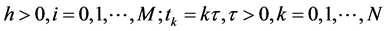，其中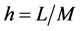分别是空间和时间步长。记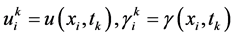，在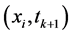处，变分数阶导数离散为：(5). (6)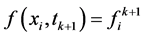，将(5)，(6)带入方程(1)，并略去高阶项得到. (7)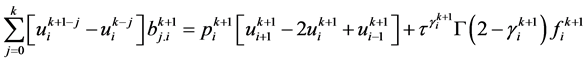. (8)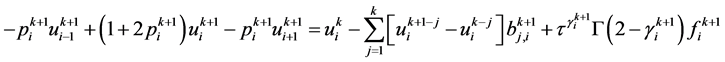. (9)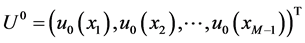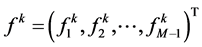，可得以矩阵形式表示的差分格式(10), (11)，这里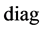表示取对角阵。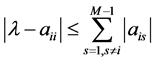,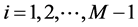.. (12)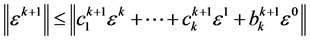. (13), (14)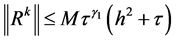,. (15), (16)

2.2. 数值算例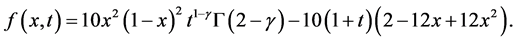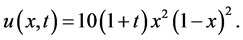3. 反问题及反演算法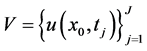, (17)Table 1. The solutions errors with space/time steps at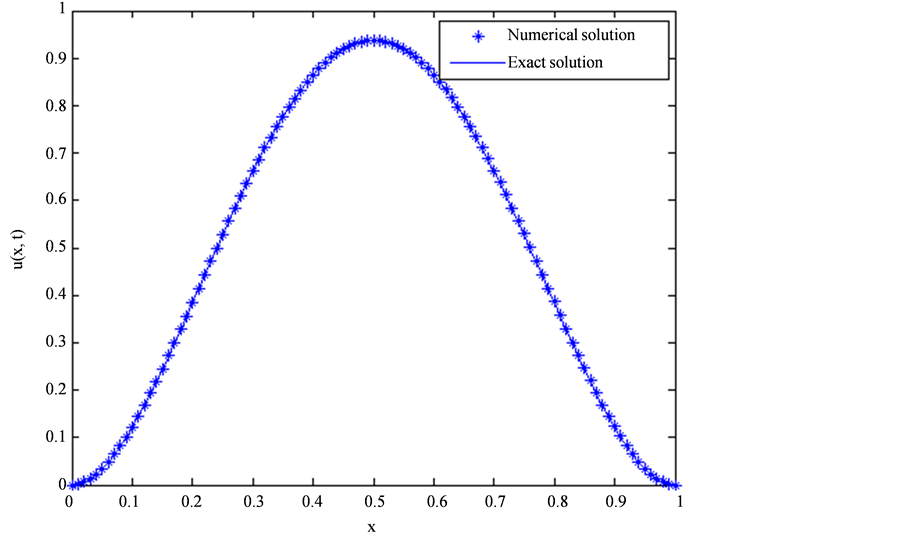Figure 1. The exact and numerical solutions at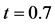for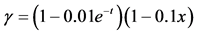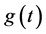的一个容许集，且设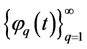的一组基。实际计算中取的有限维近似，即设, (18). (19)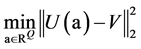. (20)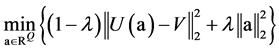, (21)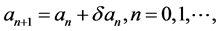(22)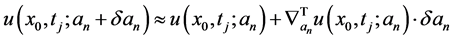,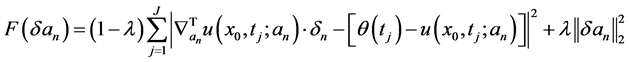.(23)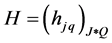,, (24)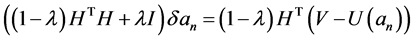, (25)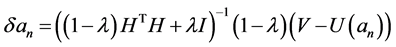. (26)

4. 数值反演, (27)

1) 先取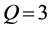，即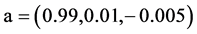，考察初始迭代对反演算法的影响。计算结果列于表2，其中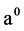表示初始迭代值，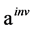表示反演解，表示反演解与真解的误差，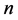为迭代次数。

2) 当在不同维数的逼近空间展开时，其有不同的表示形式。由于截断误差的存在，理论上逼近空间维数越高，则反演结果应该越精确。分别在维数为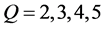的逼近空间中反演，初始迭代相应取为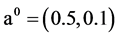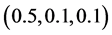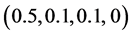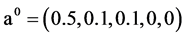，反演解与真解的图像分别绘于图2的(a)，(b)，(c)及(d)。

3) 实际问题中，附加数据往往带有某种误差，对于扰动数据实施反演算法是反问题数值方法研究的重要内容。设带扰动的附加数据表示为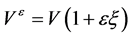, (28)

5. 结束语Table 2. The inversion results with initial iterations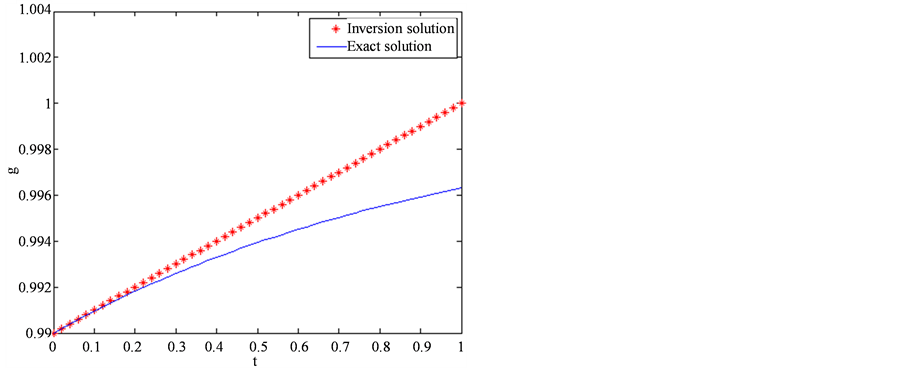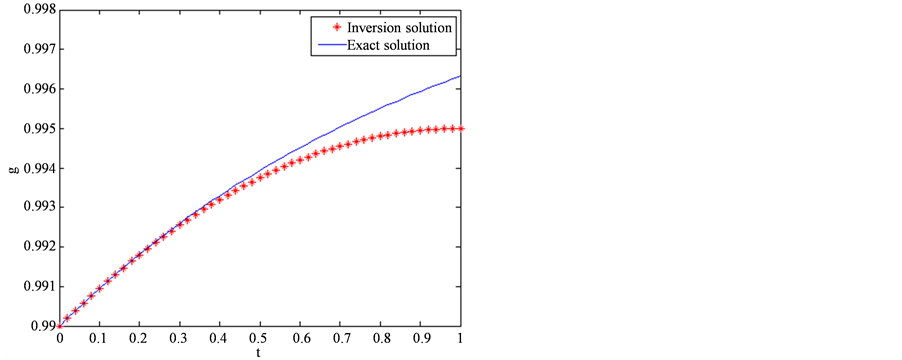(a) (b)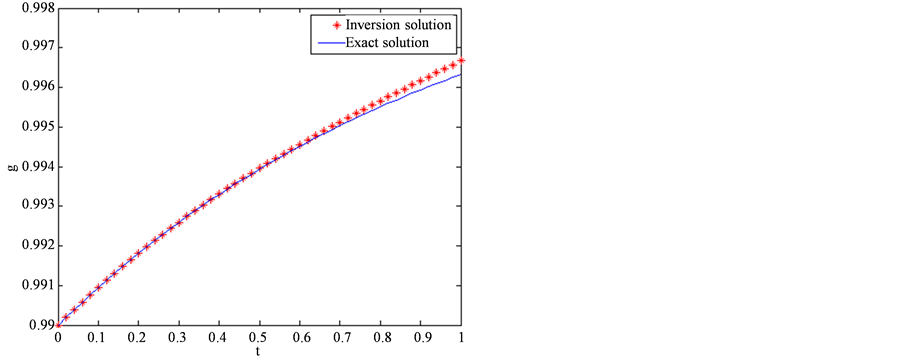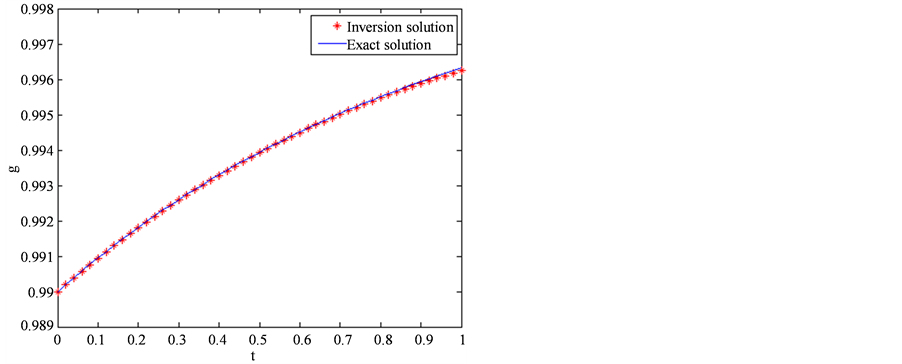(c) (d)

Figure 2. The exact and inversion solutions for the fractional order in different approximate spaces. (a) Q = 2; (b) Q = 3; (c) Q = 4; (d) Q = 5

Numerical Inversion for the Fractional Order in the Variable-Order Time-Fractional Diffusion Equation[J]. 应用数学进展, 2015, 04(04): 326-335. http://dx.doi.org/10.12677/AAM.2015.44041

1. 1. Miller, K.S. and Ross B. (1993) An Introduction to the Fractional Calculus and Fractional Differential Equations. John Wiley, New York.

2. 2. Podlubny, I. (1999) Fractional Differential Equations. Academic, San Diego.

3. 3. Kilbas, A.A., Srivastava, H.M. and Trujillo, J.J. (2006) Theory and Applications of Fractional Differential Equations. Elsevier, Amsterdam.

4. 4. 陈文, 孙洪广, 李西成. 力学与工程问题的分数阶导数建模[M]. 北京: 科学出版社, 2010.

5. 5. 郭柏灵, 蒲学科, 黄凤辉. 分数阶偏微分方程及其数值解[M]. 北京: 科学出版社, 2011.

6. 6. Samko, S.G. and Ross B. (1993) Integration and Differentiation to a Variable Fractional Order. Integral Transforms and Special Functions, 1, 277-300. http://dx.doi.org/10.1080/10652469308819027

7. 7. Ross, B. and Samko, S. (1995) Fractional Integration Operator of Variable Order in the Holder Spaces . International Journal of Mathematics and Ma-thematical Sciences, 18, 777-788. http://dx.doi.org/10.1155/S0161171295001001

8. 8. Coimbra, C.F.M. (2003) Mechanica with Variable-Order Differential Operators. Annalen der Physik, 12, 692-703. http://dx.doi.org/10.1002/andp.200310032

9. 9. Copper, G.R.J. and Rown, D.R.C. (2004) Filtering Using Variable Order Vertical Derivatives. Computers and Geosciences, 30, 455-459. http://dx.doi.org/10.1016/j.cageo.2004.03.001

10. 10. Tseng, C.C. (2006) Design of Variable and Adaptive Fractional Order FIR Differentiators. Signal Processing, 86, 2554-2566. http://dx.doi.org/10.1016/j.sigpro.2006.02.004

11. 11. Chen, C.M., Liu, F., Anh, V. and Turner, I. (2010) Numerical Schemes with High Spatial Accuracy for a Variable- Order Anomalous Subdiffusion Equation. SIAM Journal on Scientific Computing, 32, 1740-1760. http://dx.doi.org/10.1137/090771715

12. 12. Chen, C.M., Liu, F., Anh, V. and Turner, I. (2011) Numerical Simula-tion for the Variable-Order Galilei Invariant Advection Diffusion Equation with a Nonlinear Source Term. Applied Mathematics and Computation, 217, 5729-5742. http://dx.doi.org/10.1016/j.amc.2010.12.049

13. 13. Chen, C.M., Liu, F., Turner, I., Anh, V. and Chen, Y. (2013) Numerical Approximation for a Variable-Order Nonlinear Reaction-Subdiffusion Equation. Numerical Algorithms, 63, 265-290. http://dx.doi.org/10.1007/s11075-012-9622-6

14. 14. Zhang, H., Liu, F., Phanikumar, M.S. and Meer-schaert, M.M. (2013) A Novel Numerical Method for the Time Variable Fractional Order Mobile-Immobile Advec-tion-Dispersion Model. Computers and Mathematics with Applications, 66, 693-701. http://dx.doi.org/10.1016/j.camwa.2013.01.031

15. 15. 马维元, 张海东, 邵亚斌. 非线性变阶分数阶扩散方程的全隐差分格式[J]. 山东大学学报(自然科学版), 2013, 48(2): 93-97.

16. 16. 马亮亮, 刘冬兵. Coimbra变时间分数阶扩散方程–波动方程的新隐式差分法[J]. 西南师范大学学报(自然科学版), 2015, 40(3): 25-31.

17. 17. Cheng, J., Nakagawa, J., Yamamoto, M. and Yamazaki, T. (2009) Uniqueness in an Inverse Problem for a One- Dimensional Fractional Diffusion Equation. Inverse Problems, 25, Article ID: 115002. http://dx.doi.org/10.1088/0266-5611/25/11/115002

18. 18. Liu, J.J. and Yamamoto, M. (2010) A Backward Problem for the Time-Fractional Diffusion Equation. Applicable Analysis, 89, 1769-1788. http://dx.doi.org/10.1080/00036810903479731

19. 19. Sakamoto, K. and Yamamoto, M. (2011) Initial Val-ue/Boundary Value Problems for Fractional Diffusion-Wave Equations and Applications to Some Inverse Problems. Journal of Mathematical Analysis and Applications, 382, 426-447. http://dx.doi.org/10.1016/j.jmaa.2011.04.058

20. 20. Yamamoto, M. and Zhang, Y. (2012) Conditional Stability in Determining a Zeroth-Order Coefficient in a Half-Order Fractional Diffusion Equation by a Carleman Estimate. Inverse Problems, 28, Article ID: 105010. http://dx.doi.org/10.1088/0266-5611/28/10/105010

21. 21. Wei, T. and Zhang, Z.Q. (2013) Reconstruction of a Time-Dependent Source Term in a Time-Fractional Diffusion Equation. Engineering Analysis with Boundary Elements, 37, 23-31. http://dx.doi.org/10.1016/j.enganabound.2012.08.003

22. 22. Li, G.S., Zhang, D.L., Jia, X.Z. and Yamamoto, M. (2013) Simultaneous Inversion for the Space-Dependent Diffusion Coefficient and the Fractional Order in the Time-Fractional Diffusion Equation. Inverse Problems, 29, Article ID: 065014. http://dx.doi.org/10.1088/0266-5611/29/6/065014

23. 23. 贾现正, 张大利, 李功胜, 池光胜, 李慧玲. 空间–时间分数阶变系数对流扩散方程微分阶数的数值反演[J]. 计算数学, 2014, 36(2): 113-132.

24. 24. Jin, B.T. and Rundell, W. (2015) A Tutorial on Inverse Problems for Anomalous Diffusion Processes. Inverse Problems, 31, Article ID: 035003. http://dx.doi.org/10.1088/0266-5611/31/3/035003

25. 25. Li, G.S., Gu, W.J. and Jia, X.Z. (2012) Numerical Inversions for Space-Dependent Diffusion Coefficient in the Time Fractional Diffusion Equation. Journal of Inverse and Ill-Posed Problems, 20, 339-366. http://dx.doi.org/10.1515/jip-2011-0012

26. 26. Zhang, D.L., Li, G.S., Jia, X.Z. and Li, H.L. (2013) Simultaneous Inversion for Space-Dependent Diffusion Coefficient and Source Magnitude in the Fractional Diffusion Equation. Journal of Mathematics Research, 5, 65-78. http://dx.doi.org/10.5539/jmr.v5n2p65